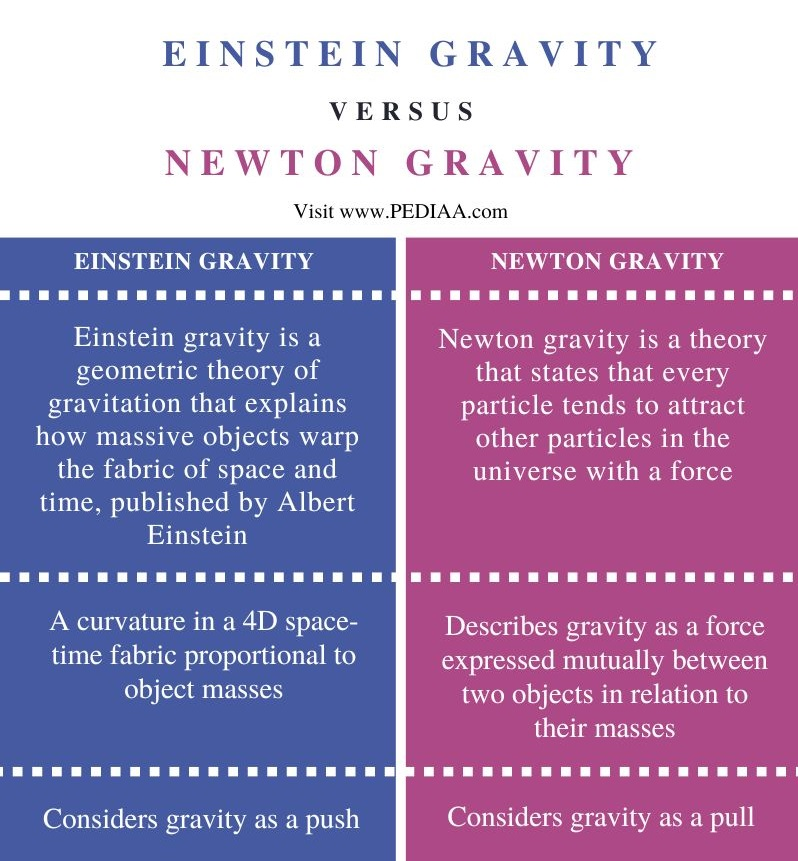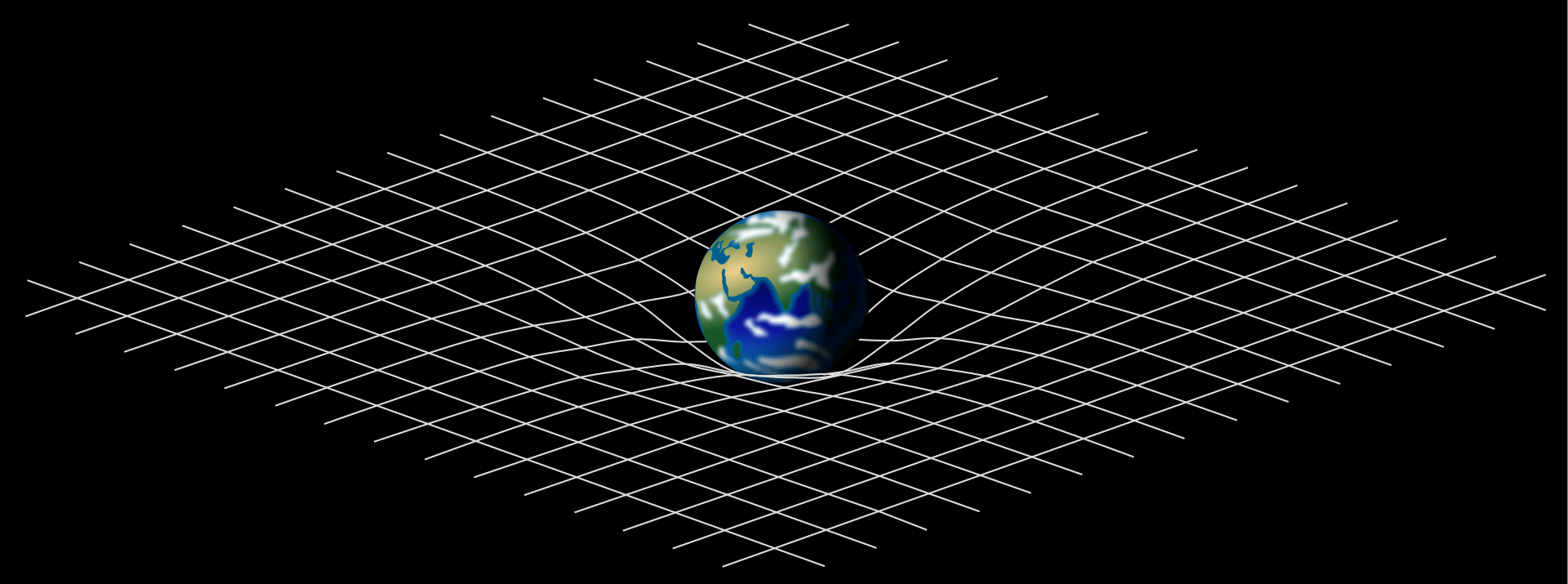# What is the Difference Between Einstein and Newton Gravity

The main difference between Einstein and Newton gravity is that Einstein described gravity as a curvature in a 4D space-time fabric proportional to the masses of objects, whereas Newton described gravity as a force between two objects based on their masses.

Gravity is a fundamental interaction (a force of attraction) that causes mutual attraction between all things with mass or energy. Gravity is a universal force, meaning it acts on all objects with mass, no matter how large or small the object is. However, compared to other fundamental forces, gravity is a relatively weak force. In brief, Einstein gravity and Newton gravity are two concepts in physics that describe the interactions between particles with masses.

### Key Areas Covered

1. What is Einstein Gravity
– Definition, Explanation
2. What is Newton Gravity
– Definition, Formula, Explanation
3. Difference Between Einstein and Newton Gravity
– Comparison of Key Differences

### Key Terms

Einstein Gravity, Newton Gravity## What is Einstein Gravity

Einstein gravity is a theory of gravity proposed by Albert Einstein in 1915. It is also known as general relativity. It is based on the idea that gravity is not a force between masses but is a result of the curvature of space-time caused by the presence of mass and energy.

Furthermore, Einstein gravity is a geometric theory of gravity, which means it describes gravity as the curvature of space-time caused by the presence of matter and energy. In this theory, the curvature of space-time is determined by the distribution of matter and energy within it.One famous prediction of Einstein gravity is the bending of light around massive objects like stars. Another prediction of Einstein gravity is the existence of gravitational waves. Moreover, these gravitational waves are the ripples in the fabric of space-time caused by the motion of massive objects.

Einsteins gravity has many applications in modern physics, including cosmology. In cosmology, it describes the large-scale structure and evolution of the universe. This is also important in the study of black holes. Einstein gravity proposes that black holes form due to the collapse of massive stars.

## What is Newton Gravity

Newton gravity is a theory that states that every particle tends to attract every other particle in the universe with a force. According to this concept, force is directly proportional to the product of masses of two particles that are subject to attraction. It is also inversely proportional to the square of the distance between their centers. Hence this is an inverse square law.

The equation for gravitation thus takes the following form.

F = G * (m1 * m2) / r^2

While F is the force of gravity between two objects, G is the gravitational constant, m1 and m2 are the masses of the two objects, and r is the distance between the centers of the two objects.Newton’s laws of universal gravitation can help to predict the nature of our solar system. Moreover, Newton’s laws are useful in sending space vehicles to outer space. It also helps to determine the path of asteroids that cross the Earth and most other celestial objects.

## Difference Between Einstein and Newton Gravity

### Definition

Einstein gravity is a geometric theory of gravitation that explains how massive objects warp the fabric of space and time, published by Albert Einstein, while Newton gravity is a theory that states that every particle tends to attract other particles in the universe with a force.

### Explanation of Gravity

Moreover, Einstein gravity is a curvature in a 4D space-time fabric proportional to object masses. Meanwhile, Newton gravity describes gravity as a force expressed mutually between two objects in relation to their masses.

### Nature

While Einstein gravity considers gravity as a push, Newton gravity considers gravity as a pull.

### Conclusion

In brief, gravity is a fundamental interaction (a force of attraction) that causes mutual attraction between all things with mass or energy. More importantly, it is a universal force. The main difference between Einstein gravity and Newton gravity is that Einstein gravity is a curvature in a 4D space-time fabric proportional to object masses, Newton gravity describes gravity as a force expressed mutually between two objects in relation to their masses.

##### Reference:

1. “13.7 Einstein’s Theory of Gravity.” Lumen Learning.
2. “Newton’s law of gravity.” Encyclopedia Brittanica.

Image Courtesy:

1. “Spacetime lattice analogy” By Mysid – Own work. Self-made in Blender & Inkscape. (CC BY-SA 3.0) via Commons Wikimedia
2. “Loi newton gravitation” By Schlecky – Own work (CC BY-SA 4.0) via Commons Wikimedia### About the Author: Hasini A

Hasini is a graduate of Applied Science with a strong background in forestry, environmental science, chemistry, and management science. She is an amateur photographer with a keen interest in exploring the wonders of nature and science.